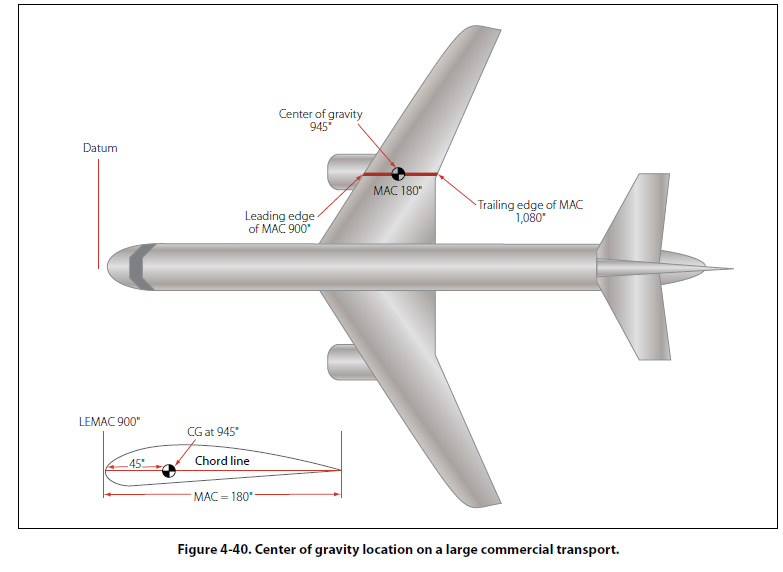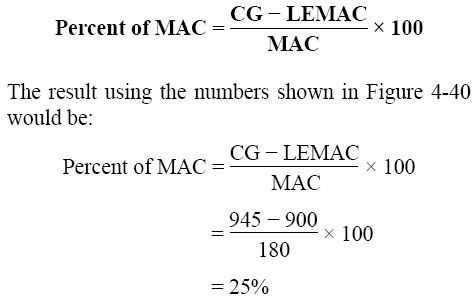Mean Aerodynamic Chord On small airplanes and on all helicopters, the center of gravity location is identified as being a specific number of inches from the datum. The center of gravity range is identified the same way. On larger airplanes, from private business jets to large jumbo jets, the center of gravity and its range are typically identified in relation to the width of the wing. The width of the wing on an airplane is known as the chord. If the leading edge and trailing edge of a wing are parallel to each other, the chord of the wing is the same along the wing’s length. Business jets and commercial transport airplanes have wings that are tapered and that are swept back, so the width of their wings is different along their entire length. The width is greatest where the wing meets the fuselage and progressively decreases toward the tip. In relation to the aerodynamics of the wing, the average length of the chord on these tapered swept-back wings is known as the mean aerodynamic chord (MAC). On these larger airplanes, the CG is identified as being at a location that is a specific percent of the mean aerodynamic chord (% MAC). For example, imagine that the MAC on a particular airplane is 100", and the CG falls 20" behind the leading edge of the MAC. That means it falls one-fifth of the way back, or at 20% of the MAC. Figure 4-40 shows a large twin-engine commercial transport airplane. The datum is forward of the nose of the airplane, and all the arms shown in the figure are being measured from that point. The center of gravity for the airplane is shown as an arm measured in inches. In the lower left corner of the figure, a cross section of the wing is shown, with the same center of gravity information being presented.To convert the center of gravity location from inches to a percent of MAC, for the airplane shown in Figure 4-40, the steps are as follows: 1. Identify the center of gravity location, in inches from the datum. 2. Identify the leading edge of the MAC (LEMAC), in inches from the datum. 3. Subtract LEMAC from the CG location. 4. Divide the difference by the length of the MAC. 5. Convert the result in decimals to a percentage by multiplying by 100. As a formula, the solution to solve for the percent of MAC would be:If the center of gravity is known in percent of MAC, and there is a need to know the CG location in inches from the datum, the conversion would be done as follows: 1. Convert the percent of MAC to a decimal by dividing by 100. 2. Multiply the decimal by the length of the MAC. 3. Add this number to LEMAC. As a formula, the solution to convert a percent of MAC to an inch value would be: G in inches = MAC % ÷ 100 × MAC + LEM AC For the airplane in Figure 4-40, if the CG was at 32.5% of the MAC, the solution would be: CG in inches = MAC % ÷ 100 × MAC + LEMAC = 32.5 ÷ 100 × 180 + 900 = 958.5
 ©AvStop Online Magazine                                                                                                                                                      Contact Us              Return To Books